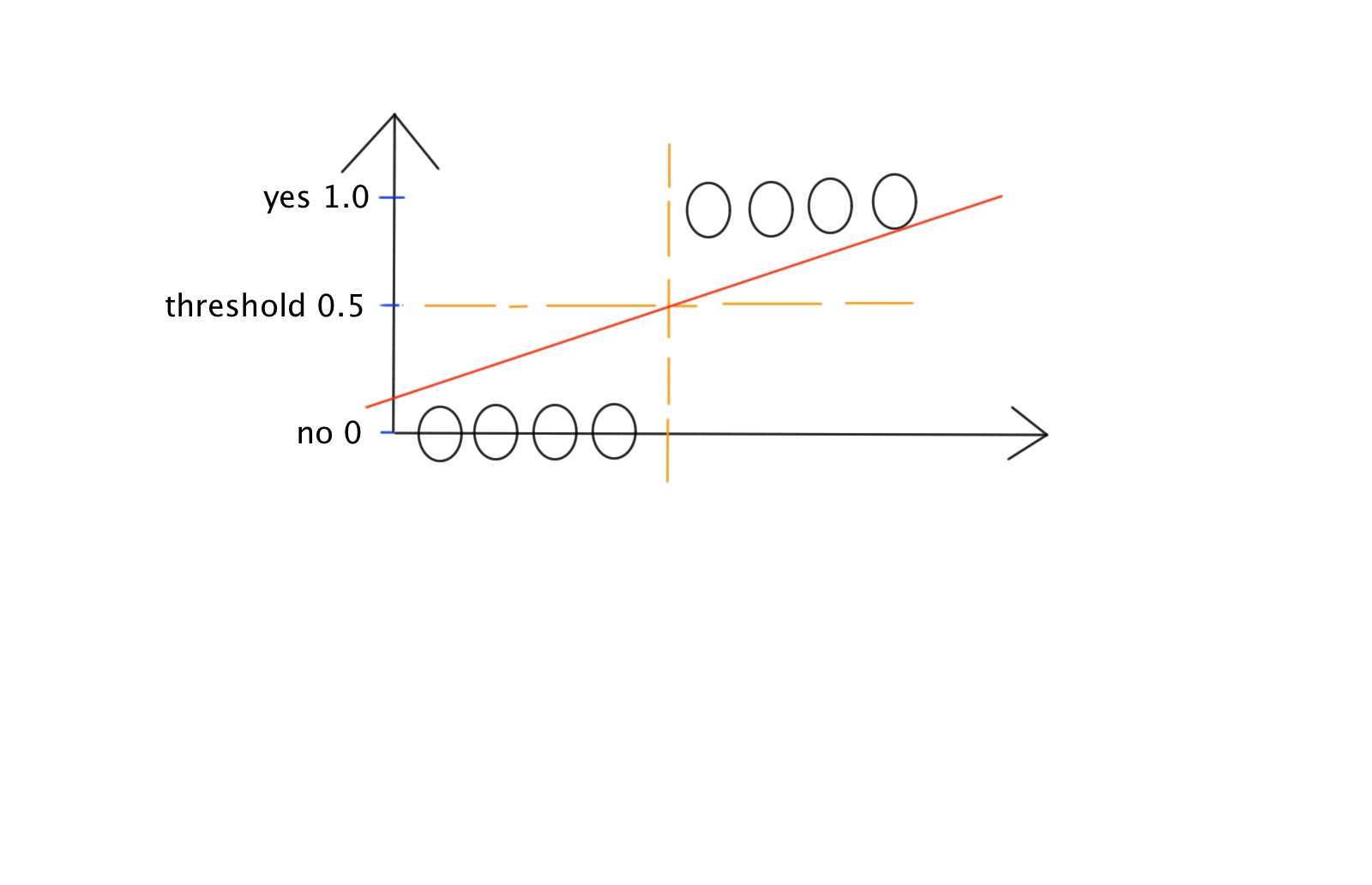## Saturday, 16 November 2013

### machine learning--03 : logistic regression

According to the open course, logistic regression is same as "classification regression", same as linear regression, it is belong to supervised machine learning algorithm, but the targets of logistic regression are digital, not analog.Following are the memo of this open course.

Example

Email : Spam/Not Spam?
Tumor : Malignant/Benign

y=1, 0
0 : "negative class"(Not Spam)
1 : "positive class""(Spam)

Question

1 : Why do we need logistic regression?Can't we just use linear regression to predict the results by setting some threshold?

A : Because the results are far from perfect. Assume we pick the threshold as 0.5graph_00

The examples of graph_00 works perfectly, but the prediction is very easy to break if we add new sample into the training set as graph_01 show.graph_01

B : the hypothesis function of linear regression can greater than 1 or less than zero.

2 : What is the model of logistic regression?

The course use sigmoid function as the model, the output range of sigmoid function is [0, 1], this is a neat feature for classification.graph_02

The output of the hypothesis is same as